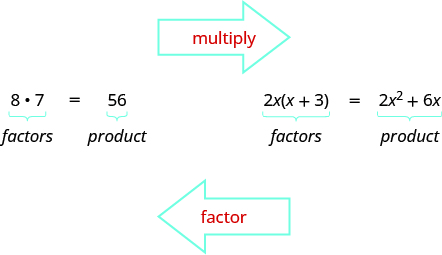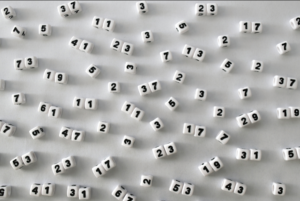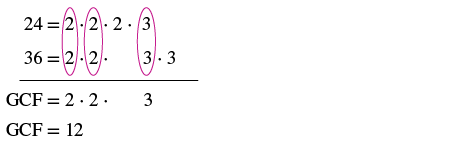## Finding the Greatest Common Factor

### Learning Outcomes

• Find the greatest common factor of multiple numbers
• Find the greatest common factor of monomials

In the section on the zero product principle, we showed that using the techniques for solving equations that we learned for linear equations did not work to solve

$t\left(5-t\right)=0$

But because the equation was written as the product of two terms, we could use the zero product principle. What if we are given a polynomial equation that is not written as a product of two terms, such as this one $2y^2+4y=0$? We can use a technique called factoring, where we try to find factors that can be divided into each term of the polynomial so it can be rewritten as a product.

In this section we will explore how to find common factors from the terms of a polynomial, and rewrite it as a product.  This technique will help us solve polynomial equations in the next section.

## Finding the Greatest Common Factor of Two Numbers

Earlier we multiplied factors together to get a product.  Factors are the building blocks of multiplication. They are the numbers that you can multiply together to produce another number: $2$ and $10$ are factors of $20$, as are  $4$ and $5$ and $1$ and $20$. To factor a number is to rewrite it as a product. $20=4\cdot5$. In algebra, we use the word factor as both a noun – something being multiplied – and as a verb – rewriting a sum or difference as a product.

Now, we will be reversing this process; we will start with a product and then break it down into its factors. Splitting a product into factors is called factoring.We also factored numbers to find the least common multiple (LCM) of two or more numbers. Now we will factor expressions and find the greatest common factor of two or more expressions. The method we use is similar to what we used to find the LCM.

When we studied fractions, we learned that the greatest common factor (GCF) of two numbers is the largest number that divides evenly into both numbers. For instance, $4$ is the GCF of $16$ and $20$ because it is the largest number that divides evenly into both $16$ and $20$.The GCF of polynomials works the same way: $4x$ is the GCF of $16x$ and $20x^2$ because it is the largest polynomial that divides evenly into both $16x$ and $20x^2$.

### Greatest Common Factor

The greatest common factor (GCF) of two or more expressions is the largest expression that is a factor of all the expressions.

First we will show how to find the greatest common factor of two numbers.Prime Numbers

To get acquainted with the idea of factoring, let’s first find the greatest common factor (GCF) of two whole numbers. The GCF of two numbers is the greatest number that is a factor of both of the numbers. Take the numbers $50$ and $30$.

$\begin{array}{l}50=10\cdot5\\30=10\cdot3\end{array}$

Their greatest common factor is $10$, since $10$ is the greatest factor that both numbers have in common.

To find the GCF of greater numbers, you can factor each number to find their prime factors, identify the prime factors they have in common, and then multiply those together.  A prime factor is similar to a prime number—it has only itself and 1 as factors. The process of breaking a number down into its prime factors is called prime factorization.

### example

Find the greatest common factor of $24$ and $36$.

Solution

 Step 1: Factor each coefficient into primes. Write all variables with exponents in expanded form. Factor $24$ and $36$.Step 2: List all factors–matching common factors in a column.In each column, circle the common factors. Circle the $2, 2$, and $3$ that are shared by both numbers.Step 3: Bring down the common factors that all expressions share. Bring down the $2, 2, 3$ and then multiply. Step 4: Multiply the factors. The GCF of $24$ and $36$ is $12$.

Notice that since the GCF is a factor of both numbers, $24$ and $36$ can be written as multiples of $12$.

$\begin{array}{c}24=12\cdot 2\\ 36=12\cdot 3\end{array}$

### Example

Find the greatest common factor of $210$ and $168$.

Because the GCF is the product of the prime factors that these numbers have in common, you know that it is a factor of both numbers. (If you want to test this, go ahead and divide both $210$ and$168$ by $42$—they are both evenly divisible by this number!)

The video that follows shows another example of finding the greatest common factor of two whole numbers.

## Greatest Common Factor of Polynomials

In the previous example, we found the greatest common factor of constants. The greatest common factor of an algebraic expression can contain variables raised to powers along with coefficients.  To factor a polynomial, you rewrite it as a product. Any integer can be written as the product of factors, and we can apply this technique to monomials or polynomials. Factoring is very helpful in simplifying and solving equations using polynomials.

Finding the greatest common factor in a set of monomials is not very different from finding the GCF of two whole numbers. The method remains the same: factor each monomial independently, look for common factors, and then multiply them to get the GCF. We summarize below a list of steps that can help you to find the greatest common factor.

### Find the greatest common factor

1. Factor each coefficient into primes. Write all variables with exponents in expanded form.
2. List all factors—matching common factors in a column. In each column, circle the common factors.
3. Bring down the common factors that all expressions share.
4. Multiply the factors.

### example

Find the greatest common factor of $5x\text{ and }15$.

### try it

In the examples so far, the greatest common factor was a constant. In the next two examples we will get variables in the greatest common factor.

### example

Find the greatest common factor of $12{x}^{2}$ and $18{x}^{3}$.

### Example

Find the greatest common factor of $25b^{3}$ and $10b^{2}$.

The monomials have the factors $5$, b, and b in common, which means their greatest common factor is $5\cdot{b}\cdot{b}$, or simply $5b^{2}$.

### example

Find the greatest common factor of $14{x}^{3},8{x}^{2},10x$.

### try it

Watch the following video to see another example of how to find the GCF of two monomials that have one variable.

We can also factor expressions that have more than one variable.

### Example

Find the greatest common factor of $81c^{3}d$ and $45c^{2}d^{2}$.

### Try It

The video that follows shows another example of finding the greatest common factor of two monomials with more than one variable.

## Contribute!

Did you have an idea for improving this content? We’d love your input.